# Chemical Reactions and Equations Class 10 Important Questions Science Chapter 1

Solved the very best collection of Chemical Reactions and Equations Class 10 Science Important Questions and Answers Chapter 1 Pdf from the latest NCERT edition books, It will help you in scoring more marks in CBSE Exams.

## Chemical Reactions and Equations Class 10 Important Questions with Answers Science Chapter 1

### Chemical Reactions and Equations Class 10 Important Questions Very Short Answer Type

Question 1.
State one basic difference between a physical change and a chemical change   (CBSE 2011)
In physical change, no new substance is formed, whereas in a chemical change, new substance(s) is/are formed.

Question 2.
What is meant by a chemical reaction?   (CBSE 2011)
The reaction representing a chemical change is called a chemical reaction.

Question 3.
Hydrogen being a highly inflammable gas and oxygen being a supporter of combustion, yet water which is a compound made up of hydrogen and oxygen is used to extinguish fire. Why?   (CBSE 2011)
It is because properties of compound (H2O) are different from properties of its constituting elements, i.e., H2 and O2.

### Chemical Reactions and Equations Class 10 Important Questions Short Answer Type

Question 1.
What happens chemically when quick lime is added to water?   (CBSE 2008)
Quick lime is CaO. On adding water to it, combination reaction takes place to give calcium hydroxide with release of large amount of heat.
CaO + H2O → Ca(OH)2

Question 2.
How will you test for the gas which is liberated when hydrochloric acid reacts with an active metal?   (CBSE 2008)
The gas liberated is hydrogen and it can be tested by taking a burning matchstick near the mouth of the test tube in which reaction is taking place. The matchstick will burn with a pop sound.

Question 3.
What is an oxidation reaction? Identify in the following reactions:
(i) the substance oxidized and
(ii) the substance reduced.
ZnO + C → Zn + CO   (CBSE 2008)
The reaction in which a substance loses hydrogen or gains oxygen is called oxidation reaction. In the given reaction C is oxidized as it gains oxygen and ZnO is reduced as it loses oxygen.

Question 4.
Give an example of photochemical reaction.  (CBSE 2008)
In black and white photography AgBr gets decomposed due to light and forms silver metal and bromine gas.

Question 5.
Give an example of a decomposition reaction. Describe an activity to illustrate such a reaction by heating.  (AI 2008)
Example of decomposition reaction:
$$\mathrm{CaCO}_{3} \stackrel{\text { heat }}{\longrightarrow} \mathrm{CaO}+\mathrm{CO}_{2}$$
Activity:
Take a test tube add CaCO3 in it and heat. CO2 gas is evolved leaving behind CaO as solid. Allow the gas to pass through lime water which turns milky and confirms CO2 gas.

Question 6.
Balance the following chemical equation:
Fe(s) + H2O(g) → Fe3O4(s) + H2(g)   (AI 2008)
3Fe(s) + 4H2O(g) → Fe3O4(s) + 4H2(g)

Question 7.
Why is respiration considered as an exothermic process?   (AI 2008)
During respiration heat is released so it is termed as an exothermic reaction.
C6H12O6 + 6O2 → 6CO2 + 6H2O + energy

Question 8.
Balance the following chemical equation:    (AI 2008C)
$$\mathrm{FeSO}_{4} \stackrel{\text { heat }}{\longrightarrow} \mathrm{Fe}_{2} \mathrm{O}_{3}+\mathrm{SO}_{2}+\mathrm{SO}_{3}$$
$$2 \mathrm{FeSO}_{4} \stackrel{\text { heat }}{\longrightarrow} \mathrm{Fe}_{2} \mathrm{O}_{3}+\mathrm{SO}_{2}+\mathrm{SO}_{3}$$

Question 9.
Balance the following chemical equation:
MnO2 + HCl → MnCl2 + Cl2 + H2O   (AI 2008)
MnO2 + 4HCl → MnCl2 + Cl2 + 2H2O

Question 10.
On what basis is a chemical equation balanced?   (Foreign 2008)
The number of atoms on left hand side of the reaction (i.e., reactants) is equal to the number of atoms on the right hand side of the equation (i.e., product).
Law of Conservation of Mass: Mass of atoms in chemical reaction can neither be created nor be destroyed, it remains the same.

Question 11.
State any two observations in an activity, which may suggest that a chemical reaction has taken place. Given examples to support your answer.   (Foreign 2008)
The following observations in an activity may suggest that a chemical reaction has taken place:

• Change in state
• Change in colour
• Formation of a precipitate
• Evolution of a gas
• Change in temperature

Question 12.
Identify the type of reaction in the following example:
Na2SO4(aq) + BaCl2(aq) → BaSO4(s) + 2NaCl(aq)     (CBSE 2008C)
It is a double displacement reaction.

Question 13.
Identify the type of reaction in the following example:
Fe(s) + CuSO4 (aq) → FeSO4(aq) + Cu(s)   (CBSE 2008C)
It is a displacement reaction. Fe displaces Cu from CuSO4.

Question 14.
Identify the type of reaction in the following example:
2H2(g) + O2(g) → 2H2O(g)   (CBSE 2008C)
It is a combination reaction.

Question 15.
Balance the given chemical equation:   (AI 2008C)
HNO3 + Ca(OH)2 → Ca(NO3)2 + H2O
2HNO3 + Ca(OH)2 → Ca(NO3)2 + 2H2O

Question 16.
Balance the given chemical equation:   (AI 2008C)
Al(s) + CuCl2(aq) → AlCl3(aq) + Cu(s)
2Al(s) + 3CuCl2(aq) → 2AlCl3(aq) + 3Cu(s).

Question 17.
Balance the following chemical equation:
$$\mathrm{Pb}\left(\mathrm{NO}_{3}\right)_{2}(\mathrm{s}) \stackrel{\text { heat }}{\longrightarrow} \mathrm{PbO}(\mathrm{s})+\mathrm{NO}_{2}(\mathrm{g})+\mathrm{O}_{2}(\mathrm{g})$$    (CBSE 2009)
$$2 \mathrm{Pb}\left(\mathrm{NO}_{3}\right)_{2} \stackrel{\text { heat }}{\longrightarrow} 2 \mathrm{PbO}(\mathrm{s})+4 \mathrm{NO}_{2}(\mathrm{g})+\mathrm{O}_{2}(\mathrm{g})$$

Question 18.
Name a reducing agent that may be used to obtain manganese from manganese dioxide.   (CBSE 2009)
Coke or carbon is used as reducing agent.

Question 19.
In electrolysis of water, why is volume of gas collected over one electrode double that of gas collected over the other electrode?   (AI 2009)
The volume of gas collected over one electrode is double than that of the gas collected over the other electrode as the ratio of H : O in water is 2 : 1.

Question 20.
What change in colour is observed when silver chloride is left exposed to sunlight?
What type of chemical reaction is this?   (Foreign 2009)
Silver turns black, the reaction is decomposition.

Question 21.
How can we know whether a reaction has taken place or not?   (Delhi 2010)
The following observations in an activity may suggest that a chemical reaction has taken place:

• Change in state
• Change in colour
• Formation of precipitate
• Evolution of a gas
• Change in temperature

Question 22.
Define a combination reaction. Give one example of a combination reaction which is also exothermic.   (CBSE 2009)
Combination reaction: It is a reaction in which two elements or compounds combine together to form a product.
e.g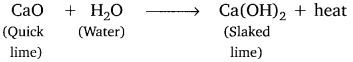Question 23.
Using a suitable chemical equation, justify that some chemical reactions are determined by:
(i) change in colour,
(ii) change in temperature.   (CBSE 2011)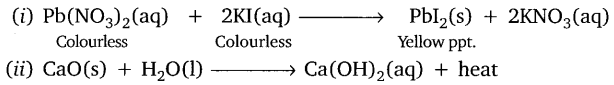Question 24.
(a) A solution of substance ‘X’ is used for white washing. What is the substance ‘X’? State the chemical reaction of ‘X’ with water.
(b) Why does the colour of copper sulphate solution change when an iron nail is dipped in it?   (CBSE 2011)
(a) ‘X’ is calcium oxide (CaO).
CaO(s) + H2O(l) → Ca(OH)2(aq) + heat
(b) It is because iron displaces copper from CuS04 to form FeS04 which is pale green.
$$\mathrm{Fe}(\mathrm{s})+\underset{\text { Blue }}{\mathrm{CuSO}_{4}}(\mathrm{aq}) \longrightarrow \underset{\text { Pale green }}{\mathrm{FeSO}_{4}(\mathrm{aq})}+\mathrm{Cu}(\mathrm{s})$$

Question 25.
Balance the following chemical equations.
(i) BaCl2 + H2SO4 → BaSO4 + HCl
(ii) Ca(OH)2 + HNO3 → Ca(NO3)2 + H2O
(iii) Pb(NO3)2 → PbO + NO2 + O2
(iv) MnO2 + HCl → MnCl2 + H2O + Cl2   (CBSE 2011)
(i) BaCl2 + H2SO4 → BaSO4 + 2HCl
(ii) Ca(OH)2 + 2HNO3 → Ca(NO3)2 + 2H2O
(iii) 2Pb(NO3)2 → 2PbO + 4NO2 + O2
(iv) MnO2 + 4HC1 → MnCl2 + 2H2O + Cl2

Question 26.
Write the balanced equation for the following reaction and identify the type of reaction in each case.
(i) Potassium bromide + Barium iodide → Potassium iodide + Barium bromide.
(ii) Hydrogen(g) + Chlorine(g) → Hydrogen chloride(g)   (CBSE 2011)
(i) 2KBr(aq) + BaI2(aq) → 2KI(aq) + BaBr2(aq)
It is a double displacement reaction.
(ii) H2(g) + Cl2(g) → 2HCl(g)
It is a combination reaction.

Question 27.
A zinc plate was put into a solution of copper sulphate kept in a glass container. It was found that blue colour of the solution gets fader and fader with the passage of time. After few days, when zinc plate was taken out of the solution, a number of holes were observed on it.
(i) State the reason for changes observed on the zinc plate.
(ii) Write the chemical equation for the reaction involved.   (CBSE 2011)
(i) It is because zinc has displaced copper from CuSO4. Zinc metal has been used to form zinc sulphate, therefore, number of holes were observed.
(ii) $$\mathrm{Zn}(\mathrm{s})+\underset{\text { Blue }}{\mathrm{CuSO}_{4}}(\mathrm{aq}) \longrightarrow \underset{\text { Colourless }}{\mathrm{ZnSO}_{4}}(\mathrm{aq})+\mathrm{Cu}(\mathrm{s})$$

Question 28.
A white salt on heating decomposes to give brown fumes and a residue is left behind.
(i) Name the salt.
(ii) Write the equation for the decomposition reaction.    (CBSE 2011)
(i) Lead nitrate is white salt.
(ii) $$2 \mathrm{Pb}\left(\mathrm{NO}_{3}\right)_{2}(\mathrm{s}) \stackrel{\text { Heat }}{\longrightarrow} 2 \mathrm{PbO}(\mathrm{s})+4 \mathrm{NO}_{2}(\mathrm{g})+\mathrm{O}_{2}(\mathrm{g})$$

Question 29.
When a solution of potassium iodide is added to a solution of lead nitrate in a test tube, a reaction takes place.
(a) What type of reaction is this?
(b) Write a balanced chemical equation to represent the above reaction.   (CBSE 2011)
Double displacement as well as precipitation reaction.
(a) Double displacement as well as precipitation reaction.
(b) $$\mathrm{Pb}\left(\mathrm{NO}_{3}\right)_{2}(\mathrm{aq})+2 \mathrm{KI}(\mathrm{as}) \longrightarrow \underset{\text { Yellow ppt. }}{\mathrm{PbI}_{2}(\mathrm{s})}+2 \mathrm{KNO}_{3}(\mathrm{aq})$$

Question 30.
Define combination reaction. Give one example of a combination reaction which is also exothermic.   (CBSE 2011)
A reaction in which two or more elements or compounds combine to form a single compound is called combination reaction.
CaO(s) + H2O(l) → Ca(OH)2(aq) + heat
It is also an exothermic reaction along with a combination reaction because heat is evolved.

Question 31.
(a) Classify the following reactions into different types.
(i) AgNO3(aq) + NaCl(aq) → AgCl(s) + NaNO3(aq)
(ii) CaO(s) + H2O(l) → Ca(OH)2(aq)
(iii) 2KClO3(S) → 2KCl(aq) + 3O2(g)
(b) Which of the above reaction(.s) is/are precipitation reaction(s)? Why is a reaction called precipitation reaction? (CBSE 2011)
(a) (i) Precipitation reaction (Double displacement reaction)
(ii) Combination reaction
(iii) Decomposition reaction
(b) Reaction (i) is a precipitation reaction because one of the product formed is insoluble in water.

Question 32.
Write balanced equations for the following mentioning the type of reaction involved.
(i) Aluminium + Bromine → Aluminium bromide
(ii) Calcium carbon → Calcium oxide + Carbon dioxide
(iii) Silver chloride → Silver + Chlorine    (CBSE 2011)
(i) 2Al(s) + 3Br2(g) → 2AlBr3(s)
(ii) CaCO3(s) → CaO(s) + CO2(g)
(in) $$2 \mathrm{AgCl}(\mathrm{s}) \stackrel{\text { Sunlight }}{\longrightarrow} 2 \mathrm{Ag}(\mathrm{s})+\mathrm{Cl}_{2}(\mathrm{g})$$

Question 33.
(a) Why is respiration considered as an exothermic reaction?
(b) Define the terms oxidation and reduction.
(c) Identify the substance that is oxidised and reduced in the following reaction.
CuO(s) + Zn(s) → Cu(s) + ZnO(s)     (CBSE 2011)
(a) It is because heat is evolved during respiration.
(c) Zn is getting oxidised, CuO is getting reduced.

Question 34.
What is meant by
(i) precipitation reaction,
(ii) exothermic reaction,
(iii) oxidation reaction?
Write balanced chemical equations for an example of each.       (CBSE 2011)
(i) Precipitation reaction: The reaction in which two compounds exchange their ions and the product formed is insoluble in water is called precipitation reaction.
AgNO3(aq) + KI(aq) → Agl(s) + KNO3(aq)
(ii) Exothermic reaction: The reaction in which heat is evolved is known as exothermic reaction.
C + O2 → CO2 + heat
(iii) Oxidation reaction: The reaction in which O2 is added or H2 is removed or loss of electrons takes place is called oxidation reaction.
2Cu + O2 → 2CuO

Question 35.
You might have noted that when copper powder is heated in a china dish, the surface of copper powder becomes coated with a black colour substance.
(i) How has this black coloured substance formed?
(ii) What is that black substance?
(iii) Write the chemical equation of the reaction that takes place.     (CBSE 2011)
(i) Copper reacts with oxygen to form copper oxide which is black, i.e., oxidation of copper takes place
(ii) Copper oxide
(iii) 2Cu(s) + O2(g) → 2CuO(s)

Question 36.
Write balanced chemical equations for the following reactions.
(i) Silver bromide on exposure to sunlight decomposes into silver and bromine,
(ii) Sodium metal reacts with water to form sodium hydroxide and hydrogen gas.     (CBSE 2012)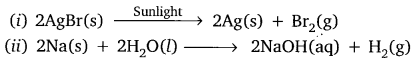Question 37.
Identify the type of reactions(s) in the following equations.
(i) CH4 + 2O2 → CO2 + 2H2O
(ii) Pb(NO3)2 + 2Kl → Pbl2 + 2KNO3
(iii) CaO + H2O → Ca(OH)2
(vi) CuSO4 + Zn → ZnSO4 + Cu     (CBSE 2012)
(i) Combustion reaction and oxidation reaction.
(ii) Double displacement reaction and precipitation reaction.
(iii) Combination reaction.
(iv) Displacement reaction.

Question 38.
Write balanced equation for the reaction between magnesium and hydrochloric acid. Name the product obtained, identify the type of reaction. (CBSE 2012)
Mg(s) + 2HCl(dil.) → MgCl2(aq) + H2(g)
The product formed is magnesium chloride and hydrogen gas.
It is a displacement reaction.

Question 39.
Describe an activity to observe what happens when quicklime is added to water taken in a beaker. State two important observations and name the type of reaction taking place.   (CBSE 2012)
Aim: To observe what happens when quicklime is added to water taken in a beaker.
Materials Required: Quicklime (calcium oxide), water, beaker.
Procedure:
1. Take 5g of calcium oxide in a beaker.
2. Add water to it slowly.
3. Touch the beaker.
4. Note down the observations.Observation:
Calcium oxide reacts with water vigorously to form calcium hydroxide with the evolution of heat.
Chemical Reaction:Conclusion:
The reaction between CaO (calcium oxide) and H2O is a combination reaction. It is an exothermic process because heat is evolved.

Question 40.
What is the colour of ferrous sulphate crystals? How does this colour change after heating?   (CBSE 2012)
The colour of ferrous sulphate is pale green. The colour changes to reddish brown on heating due to formation of iron (III) oxide.

Question 41.
Why does the colour of copper sulphate solution change when an iron nail is dipped in it? Write two observations. (CBSE 2012)
It is because displacement reaction takes place. Iron displaces copper from copper sulphate solution and forms pale green colured solution of FeSO4 and reddish brown copper metal gets deposited.
Fe(s) + CuSO4(aq) → FeSO4(aq) + Cu(s)

Question 42.
Translate the following statement into chemical equation and then balance it. Barium chloride reacts with aluminium sulphate to give aluminium chloride and a precipitate of barium sulphate. State the two types in which this reaction can be classified.   (CBSE 2012)
3BaCl2(aq) + Al2(SO4)3(aq) → 3BaSO4(s) + 2AlCl3(aq)
It can be classified as double displacement as well as precipitation reaction.

Question 43.
Why are decomposition reactions called the opposite of combination reactions? Write equations for these reactions. (CBSE 2012)

Question 44.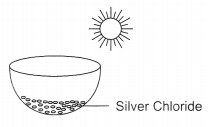The following diagram displays a chemical reaction.
Observe carefully and answer the following questions
(a) Identify the type of chemical reaction that will take place and define it. How will the colour of the salt change?
(b) Write the chemical equation of the reaction that takes place.
(c) Mention one commercial use of this salt.   (CBSE 2012)
(a) Photochemical decomposition reaction: Those reactions in which a compound breaks down into simple substances in presence of light are called photochemical decomposition reaction. The colour of salt will change from white to grey.
(b) $$2 \mathrm{AgCl}(\mathrm{s}) \stackrel{\text { Sunlight }}{\longrightarrow} 2 \mathrm{Ag}(\mathrm{s})+\mathrm{Cl}_{2}(\mathrm{g})$$
(c) Silver chloride is used in photography.

Question 45.
What is rancidity? Mention any two ways by which rancidity can be prevented.   (CBSE 2012)
The process in which taste and smell of food containing oils or fats gets spoiled is called rancidity. It happens due to oxidation.

Prevention from rancidity:

1. Antioxidants are added to fatty acids to prevent oxidation, e.g., chips are packed in presence of nitrogen gas which prevents spoilage by oxidation.
2. Food should be kept in airtight container in refrigerator.

Question 46.
Write balanced chemical equation for the reactions that take place during respiration. Identify the type of combination reaction that takes place during this process and justify the name. Give one more example of this type of reaction.    (CBSE 2012)
C6H12O6 + 6O2 → 6CO2 + 6H2O + heat
It is an exothermic combination reaction because heat is evolved.
CH4(g) + 2O2(g) → CO2(g) + 2H2O
Combustion of methane is another example of exothermic combination reaction.

Question 47.
What is redox reaction? Identify the substance oxidised and the substance reduced in the following reactions.
(i) 2PbO + C → 2Pb + CO2
(ii) MnO2 + 4HCl → MnCl2 + 2H2O + Cl2    (CBSE 2012)
Those reactions in which oxidation and reduction takes place simultaneously are called redox reactions.
(i) PbO getting reduced and C is getting oxidsed.
(ii) MnO2 is getting reduced and HCl is getting oxidised.

Question 48.
Write the balanced chemical equations for the following reactions and identify the type of reaction in each case.
Thermite reaction, iron (III) oxide reacts with aluminium and gives molten iron and aluminium oxide  (CBSE 2012)It is a displacement reaction because aluminium is more reactive then iron and displaces it. The iron melted in here is used in joining the railway tracks.

Question 49.
Which products will be obtained when lead nitrate is heated simply. Write balanced chemical equation for the reaction. State the type of chemical reaction that occur in the change.     (CBSE 2013)
Lead monoxide, nitrogen dioxide and oxygen gas will be liberated.
$$2 \mathrm{Pb}\left(\mathrm{NO}_{3}\right)_{2}(\mathrm{s}) \stackrel{\text { heat }}{\longrightarrow} 2 \mathrm{PbO}(\mathrm{s})+4 \mathrm{NO}_{2}(\mathrm{g})+\mathrm{O}_{2}(\mathrm{g})$$
It is thermal decomposition reaction.

Question 50.
What is meant by skeletal type chemical equation? What does it represent? Using the equation for electrolytic decomposition of water, differentiate between a skeletal chemical equation and a balanced chemical equation. (CBSE 2013)
The equation in which reactants and products formed are shown by their chemical formulae without considering the balancing of atoms is called skeletal type equation. It represents the reactants and products that are involved in the reaction.
H2O → H2 + O2 (skeletal equation)
2H2O → 2H2 + O2 (Balanced chemical equation)

Question 51.
What is observed when a solution of potassium iodide solution is added to a solution of lead nitrate? Name the type of reaction. Write a balanced chemical equation to represent the above chemical reaction.    (CBSE 2014)
Yellow precipitate of lead iodide is formed. It is a precipitation reaction.
Pb(NO3)2(aq) + 2KI(aq) → PbI2(s) + 2KNO3(aq)
It is also called double displacement reaction.

Question 52.
Write chemical equation of reactions taking place when carried out with the help of
(a) Iron reacts with steam
(b) Magnesium reacts with dil HCl
(c) Copper is heated in air.     (CBSE 2014)
(a) 3Fe(s) + 4H2O(g) → Fe3O4(s) + 4H2(g)
(b) Mg(s) + 2HCl(aq) → MgCl2(aq) + H2(g)
(c) $$2 \mathrm{Cu}(\mathrm{s})+\mathrm{O}_{2}(\mathrm{g}) \stackrel{\text { heat }}{\longrightarrow} 2 \mathrm{CuO}(\mathrm{s})$$

Question 53.
“We need to balance a skeletal chemical equation.” Give reason to justify the statement.    (CBSE 2015)
Skeletal chemical equations are unbalanced. We need to balance chemical equation because of law of conservation of mass. It states that ‘matter can neither be created nor be destroyed’. Therefore, each and every atom involved in chemical equation must be balanced.

Question 54.
Giving an example list two information which make a chemical equation more useful (informative)     (CBSE 2015)
(i) Physical state of reactants must be mentioned, e.g.,
2H2(g) + O2(g) → 2H2O(l)
(ii) Condition in which reaction takes place are written on the arrow head, e.g.,Question 55.
Consider the following chemical reaction
X + Barium chloride → Y + Sodium chloride
(a) Identify ‘X’ and ‘Y
(b) The type of reaction (CBSE 2015)
(a) ‘X’ is Na2SO4 and Y is BaSO4
(b) The type of reaction
Na2SO4 + BaCl2 → BaSO4 + 2NaCl
(White ppt)
The reaction is precipitation reaction. It is also a double displacement reaction

Question 56.
Name the reducing agent from the following reaction:
3MnO2 + 4Al → 3Mn + 2Al2O3
State which is more reactive, Mn or Al and why?     (CBSE 2015)
‘Al’ is more reactive than Mn as ‘Al’ displaces Mn from its oxide.

Question 57.
(i) Write a balanced chemical equation for process of photosynthesis.
(ii) When do desert plants take up carbon dioxide and perform photosynthesis?        (CBSE 2015)
(I) $$6 \mathrm{CO}_{2}(\mathrm{g})+6 \mathrm{H}_{2} \mathrm{O}(\mathrm{l}) \stackrel{\text { Sunlight }}{\longrightarrow} \mathrm{C}_{6} \mathrm{H}_{12} \mathrm{O}_{6}(\mathrm{s})+6 \mathrm{O}_{2}(\mathrm{g})$$
(ii) In desert plants the stomata are open at night. They take CO2 at night and is
stored in the from of acid and is used during day time for photosynthesis.

Question 58.
Name the type of chemical reaction represented by the following equation:
(i) CaO + H2O → Ca(OH)2
(ii) 3BaCl2 + Al2(SO2)3 → 3BaSO4 + 2AlCl3
(iii) 2FeSO4 → Fe2O3 + SO2 + SO3          (CBSE 2015)
(i) Combination reaction
(ii) Double displacement reaction (Precipitation reaction)
(iii) Decomposition reaction.

Question 59.
Write the chemical equation of the reaction in which the following changes have taken place with an example of each:
(i) Change in colour
(ii) Change in temperature
(iii) Formation of precipitate    (CBSE 2015)
(i) Cu(s) + 2AgNO3(aq) → Cu(NO3)2(aq) + 2Ag(s)
The solution will become blue in colour and shiny silver metal will be deposited.
(ii) NaOH + HCl → NaCl + H2O + heat
The temperature will increase because heat will be evolved.
(iii) Pb(NO3)2(aq) + 2KI(aq) → Pbl2(s) + 2KNO3(aq)
Yellow precipitate of Pbl2 will be formed

Question 60.
State the type of chemical reactions and chemical equations that take place in the following:
(i) Magnesium wire is burnt in air.
(ii) Electric current is passed through water,
(iii) Ammonia and hydrogen chloride gases are mixed.     (CBSE 2015)
(i) 2Mg(s) + O2(g) → 2MgO(s)
Combination reaction (Redox reaction).
(ii) $$2 \mathrm{H}_{2} \mathrm{O}(\mathrm{l}) \stackrel{\text { electrolysis }}{\longrightarrow} 2 \mathrm{H}_{2}(\mathrm{g})+\mathrm{O}_{2}(\mathrm{g})$$
Electrical decomposition reaction.
(iii) NH3(g) + HCl(g) → NH4Cl(s)
Combination reaction.

Question 61.
(a) Write the essential condition for the following reaction to take place:
2AgBr → 2Ag + Br2
Write one application of this reaction.
(b) Complete the following chemical equation
$$2 \mathrm{FeSO}_{4} \longrightarrow \stackrel{\Delta}{\longrightarrow} \mathrm{Fe}_{2} \mathrm{O}_{3}+\ldots+\ldots$$
(c) What happens when water is added to quick lime? Write chemical equation. (CBSE 2015)
(a) $$2 \mathrm{AgBr} \stackrel{\text { Sunlight }}{\longrightarrow} 2 \mathrm{Ag}+\mathrm{Br}_{2}$$
This reaction used in photography
(b) $$2 \mathrm{FeSO}_{4} \stackrel{\text { heat }}{\longrightarrow} \mathrm{Fe}_{2} \mathrm{O}_{3}+\mathrm{SO}_{2}+\mathrm{SO}_{3}$$
(c) Slacked lime is formed with hissing sound and lot of heat is envolved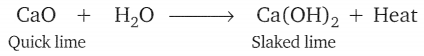Question 62.
2g of ferrous sulphate crystals are heated in a dry boiling tube.
(i) List any two observations
(ii) Name the type of chemical reaction taking place.
(iii) Write the chemical equation for the reaction. (CBSE 2015)
(i) Green colour of FeSO4 disappears and reddish brown solid is formed.
Smell of burning sulphur.
(ii) Decomposition reaction
(iii) $$2 \mathrm{FeSO}_{4}(\mathrm{s}) \stackrel{\text { heat }}{\longrightarrow} \mathrm{Fe}_{2} \mathrm{O}_{3}(\mathrm{s})+\mathrm{SO}_{2}(\mathrm{g})+\mathrm{SO}_{3}(\mathrm{g})$$

### Chemical Reactions and Equations Class 10 Important Questions Long Answer Type

Question 1.
Solid calcium oxide was taken in a container and water was added slowly to it.    (AI 2008C)(i) State the two observations made in the experiment.
(ii) Write the name and chemical formula of the product formed.
(i) Calcium oxide reacts vigorously with water to produce slaked lime and large amount of heat is released.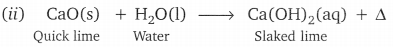Question 2.
What is an oxidation reaction? Give an example of oxidation reaction. Is oxidation an exothermic or an endothermic reaction? (CBSE 2009)
The reaction in which oxygen is added or hydrogen is removed is called oxidation reaction.
$$\text { e.g., } 2 \mathrm{Cu}+\mathrm{O}_{2} \stackrel{\text { heat }}{\longrightarrow} 2 \mathrm{CuO}$$
It is an endothermic reaction.

Question 3.
(i) What is observed when a solution of potassium iodide is added to a solution of lead nitrate taken in a test tube?
(ii) What type of reaction is this?
(iii) Write a balanced chemical equation to represent the above reaction.     (AI 2009)
(i) Yellow coloured precipitate is formed due to formation of lead iodide.
(ii) It is a double displacement reaction.
(iii) 2KI + Pb(NO3)2 → 2KNO3 + Pbl2

Question 4.
Distinguish between an exothermic and an endothermic reaction. Amongst the following reactions, identify the exothermic and the endothermic reaction.
(i) Heating coal in air to form carbon dioxide.
(ii) Heating lime-stone in a lime kiln to form quick lime.       (Foreign 2009)

 Exothermic Reaction Endothermic Reaction The reaction in which heat is released. The reaction in which heat is absorbed.

(i) Exothermic
(ii) Endothermic

Question 5.
Distinguish between a displacement reaction and a double displacement reaction. Identify the displacement and the double displacement reaction from the following reactions.
(i) HCl(aq) + NaOH(aq) → NaCl(aq) + H2O(l)
(ii) Fe(s) + CuSO4(aq) → FeSO4(aq) + Cu(s)     (Foreign 2009)

 Displacement Reaction Double Displacement Reaction The reaction in which more reactive metal displaces less reactive metal. The reaction in which two different atoms or group of atoms exchange for each other.

(i) It is a double displacement reaction.
(ii) It is a displacement reaction.

Question 6.
(a) Write one example for each of decomposition reaction carried out with help of
(i) Electricity
(ii) Heat
(iii) Light
(b) Which of the following statements is correct and why copper can displace silver from silver nitrate and silver can displace copper from copper sulphate solution.        (CBSE 2014)(b) Copper can displace silver from AgN03 because copper is more reactive than Ag
Cu + 2AgNO3(ag) → Cu(NO3)2(aq) + 2Ag(s)

Question 7.
(a) Define a balanced chemical equation. Why should an equation be balanced?
(b) Write the balanced chemical equation for the following reaction:
(i) Phosphorus burns in presence of chlorine to form phosphorus pentachloride.
(ii) Burning of natural gas.
(iii) The process of respiration.      (CBSE 2015)
(a) Balanced chemical equation has an equal number of atoms of different elements in the reactants and products. According to law of conservation of mass, matter can neither be created nor be destroyed in a chemical reaction.
(b) (i) P4(s) + 10Cl2(g) → 4PCl5(S)
(ii) CH4(g) + 2O2(g) → CO2(g) + 2H2O(g) + heat energy
(iii) C6H12O6(S) + 6O2(g) → 6CO2(aq) + 6H2O(l) + energy

Question 8.
(a) Explain two ways by which food industries prevent rancidity.
(b) Discuss the importance of decompostion reaction in metal industry with three points. (CBSE 2015)